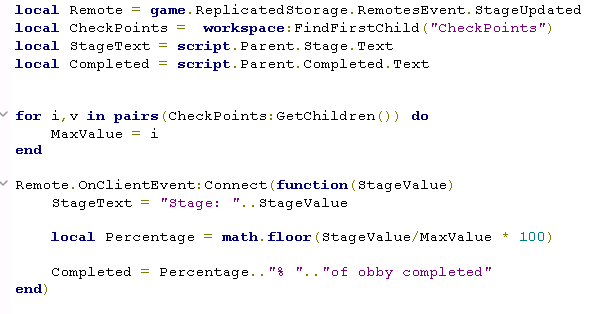# How to make a percentage?

I’m working on a project and at the moment I need to use a percentage, but I don’t know how to do it, if you can help me I’ll be happy!

Example:

``````local Value = 10
local MaxValue = 230
local Text = script.Parent.Text

Text = "Percentage" of obby completed!
``````

can you create an example if possible?

10 Likes

Could you please elaborate? Thank you.

You can’t really use % so you just will have to use decimals like 50% = 0.5

So if you need 50% of 100 then you do (0.5 * 100)

1 Like

If you’re asking how to calculate a percentage it is literally just the value divided by the max value.

But as @misthero21 said it is going to come out as a decimal value, so if you want to format it you would have to multiply it by 100.

``````local Ratio = Value / MaxValue
Ratio = math.floor(ratio * 100 + 0.5) -- Round to nearest whole number.
Text = Ratio.."% of obby completed!"
``````
4 Likes

If I get you correct, you want a script that tells you how much you have completed an obby?

Thinking that percentage can’t go above 100% (If we don’t mean that you can complete more than the stage itself, which shouldn’t be possible) you should be able to get the percentage by dividing the Players Value with the Maximum Value.

Example Script:

``````local PlayerValue = 10
local MaxValue = 230
local Text = script.Parent.Text

local Percentage = (PlayerValue / MaxValue) * 100

Text = "The Player has completed ".. math.floor(Percentage) .. " of the Obby!"
``````

If you want this to stay up to date, just put it in a while true do loop like this:

``````while true do
wait()
local PlayerValue = 10
local MaxValue = 230
local Text = script.Parent.Text

local Percentage = (PlayerValue / MaxValue) * 100

Text = "The Player has completed ".. math.floor(Percentage) .. " of the Obby!"
end
``````

EDIT: Just saw that Blokav above wrote something similair while I was typing. It also works

3 Likes

Busy loops like these are not good practice; it’s preferable OP updates based on some event, whether manually triggered or through a Roblox connection.

2 Likes

already made a loop using an event that is triggered when DataStore2 is updated, thanks for the tip

Just for fun, you can use string’s format function too:

``````local text = ("%i%% of obby completed!"):format(value / maxValue * 100)
``````

The “%i” is replaced with an integer number, thus a non-integer will just get its decimal cut off (essentially flooring the value). The “%%” just escapes the “%” so it will appear as-is and not get formatted.

3 Likes

this will help me a lot! thanks.

I don’t know why, but it seems that the script is not being read by the client, and it doesn’t show any errors in the script!In this script you are actually initializing “StageText” and “Completed” as string variables; you can’t directly reference properties of instances like what you seem to be trying to do.
Try initializing them as this:

``````local StageText = script.Parent.Stage
local Completed = script.Parent.Completed
``````

Then update them like this:

``````StageText.Text = "Stage: "..StageValue
local Percentage = math.floor(StageValue / MaxValue * 100)
Completed.Text = Percentage.."% of obby completed"
``````
9 Likes

is there any way I can get just two decimals? for example: 51.23667 to 51.23

You can round to the n’th digit by multiplying by 10^n, flooring, then dividing back:

``````Percentage = StageValue / MaxValue * 100
Percentage = math.floor(Percentage * 100) / 100
``````

if my Stage Value starts with 1 how do I get the percentage to be zero when I start? sorry the inconvenience is that I am very confused

You would have to offset them by one then.

``````Percentage = (StageValue - 1) / (MaxValue - 1) * 100
``````

When StageValue == 1, Percentage would be zero
When StageValue == MaxValue, Percentage would be 100Could you show the exact line of code where you perform this calculation in that it results in a negative value? This should not be the case when you’re offsetting the value.

Be sure to also include updated code lines when something doesn’t work so we can build off your progress and see what the issue may be, thus allowing us to better help you.

``````Remote.OnClientEvent:Connect(function(StageValue)
StageText.Text = "Stage: "..StageValue

local Percentage = math.floor(StageValue-1/(MaxValue-1) * 100) -- here

Completed.Text = Percentage.."% ".."of obby completed"
end)``````

realized my mistake lol I put:
StageValue-1/(MaxValue-1) * 100
rather than:
(StageValue-1)/(MaxValue-1) * 100

Your order of operations is starting to look confusing. I like to organize these kinds of expressions with parentheses. It ensures that operations are done in the order they are supposed to and also makes it more readable.

What the VM sees is this:

MaxValue - 1
1 / (MaxValue - 1)
(1 / (MaxValue - 1)) * 100
StageValue - ((1 / (MaxValue - 1)) * 100)

Which looks nothing like what we’re trying to do.

``````local Percentage = math.floor((StageValue-1)/(MaxValue-1) * 100)
``````
1 Like